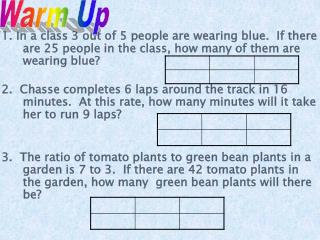Download PresentationWarm Up

# Warm Up - PowerPoint PPT PresentationDownload Presentation## Warm Up

- - - - - - - - - - - - - - - - - - - - - - - - - - - E N D - - - - - - - - - - - - - - - - - - - - - - - - - - -
##### Presentation Transcript

1. Warm Up 1. In a class 3 out of 5 people are wearing blue. If there are 25 people in the class, how many of them are wearing blue? 2. Chasse completes 6 laps around the track in 16 minutes. At this rate, how many minutes will it take her to run 9 laps? 3. The ratio of tomato plants to green bean plants in a garden is 7 to 3. If there are 42 tomato plants in the garden, how many green bean plants will there be?

2. WarM Up Stephanie and Tamara both started running a race at 8:15 A.M. Stephanie finished the race in 4hours 30minutes. Tamara finished the race in 1hour 15minutes after Stephanie did. At what time did Tamara finish the race? A bus arrived at its destination at 2:15 P.M. If the trip took 3 hours and 50 minutes, at what time did the bus depart?

3. Find and interpret the probability of a simple event. • outcomes • simple event • probability • random • complementary events

4. Interactive Lab: Probability and Area

5. Answer: Find Probability There are six equally likely outcomes on the spinner shown. Find the probability of landing on 1. There is one section of the spinner labeled 1.

6. A. B. C. D. A number cube is rolled. Find the probability of rolling a 4. • A • B • C • D

7. Answer: Find Probability There are six equally likely outcomes on the spinner shown. Find the probability of landing on 2 or 4. The word or indicates that the number of favorable outcomes needs to include the section labeled 2 and the section labeled 4. There is one section of the spinner labeled 2 and one section labeled 4.

8. A. B. C. D. A number cube is rolled. Find the probability of rolling a number greater than 3.

9. At a school carnival, Sarosha’s little sister wants to play the Duck Pond game. Each player wears a blindfold and chooses a duck from a tub of water. The player then wins the prize written on the bottom of the duck. There are 3 ducks with doll, 3 ducks with jump rope, 4 ducks with coloring book, and 6 ducks with bubble gum. What is the probability that Sarosha’s sister will choose a duck printed with bubble gum?

10. Find Probability of the Complement There are six equally likely outcomes on the spinner shown. The spinner is spun once. Find the probability of not landing on 6. The probability of not landing on 6 and the probability of landing on 6 are complementary. So, the sum of the probabilities is 1.

11. Answer: Find Probability of the Complement

12. A store is having a Wheel of Savings sale. They allow each customer to spin their wheel to earn a discount off of their purchase. What is the probability of getting 15% off? 10 % 15 % 15 % 50 % 10 % 10 % 15 % 20 % 15 % 20 %

13. A. B. C. D. A number cube is rolled. Find the probability of not rolling an even number. • A • B • C • D

14. Lesson 4 Ex4 Find Probability of the Complement SPORTSA sportscaster predicted that the Tigers had a 75% chance of winning tonight. Describe the complement of this event and find its probability. The complement of winning is not winning. The sum of the probabilities is 100%. Answer: So, the probability that the Tigers will not win tonight is 25%.

15. SLEEPOVERCelia guesses the probability that her parents will allow her to sleep over at her best friend’s house tonight is 55%. What is the probability that Celia will not be allowed to sleep over? • A • B • C • D A. 20% B. 25% C. 45% D. 50%

16. If you spin the spinner shown below, what is the probability of landing on pink?